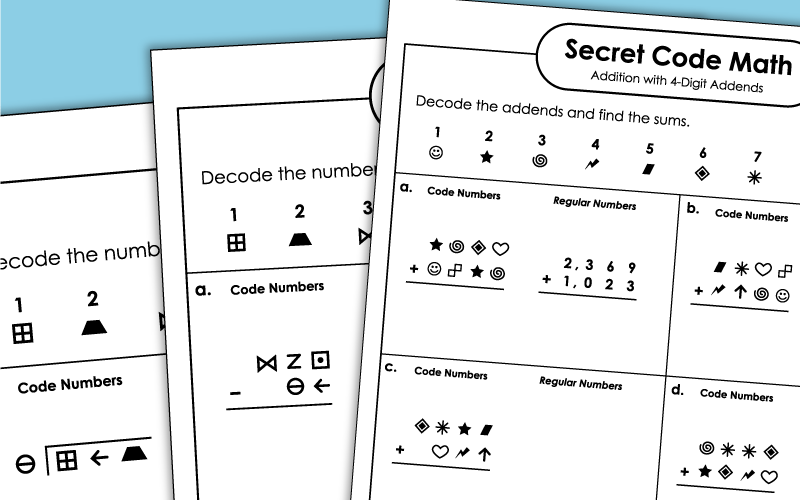# Secret Code Math

The printable "Secret Code Math" worksheets below cover dozens of different math topics, including addition, multiplication, division, and subtraction. Students first decode the numbers in the problems, then they solve.On this worksheet, students will decipher the code to create 3-digit addition problems. Then they'll solve each one.
2nd through 4th Grades
To solve these addition problems, students will first need to decipher the symbol addends.
2nd through 4th Grades
This column addition worksheet has three addends. Each one has 2 or 3 digits.
2nd through 4th Grades
These coded problems have up to 4 addends, with 2, 3, or 4 digits in each.
3rd through 5th Grades

## Subtraction

These secret code math subtraction problems each have 2-digits. Students do not borrow or regroup to solve.
1st and 2nd Grades
Students will need to borrow/regroup to solve the subtraction problems on this sheet.
1st through 3rd Grades
After decoding the numbers using the key, students will subtract to find the differences.
2nd through 4th Grades
These 3-digit subtraction problems require students to borrow across zero. (example: 400-126)
2nd through 4th Grades
Translate the picto-code numbers into "regular numbers" and then solve. All problems require students to subtract 4-digit numbers across zero. (example: 5,000-2,614)
3rd and 4th Grades

## Rounding

Decode the numbers, then round each one to the nearest ten. (example: 264 rounds to 260)
2nd through 4th Grades
On this one, children will figure out the numbers and then round to the nearest thousand.
4th through 6th Grades

## Comparing Numbers

Decode sets of symbols into numbers. Compare each group with "greater than," "less than," or "equal to."
1st through 3rd Grades

## Multiplication

Decode the factors. Then write the product for each basic multiplication fact, up to 10x10.
3rd and 4th Grades
First decode the numbers using the key at the top of the page. Then find the product for each pair of numbers.
(example: 54 x 6)
3rd and 4th Grades
Find the products by multiplying 4-digit numbers by 1-digit numbers.
(example: 3,478 x 4)
4th and 5th Grades
Here you'll multiply pairs of double-digit numbers together.
(example: 84 x 51)
4th and 5th Grades
Multiply 3-digit numbers by 2-digit numbers in this cypher-code activity.
4th through 6th Grades

## Division

Convert the pictures into numbers, then write the quotient for each basic division fact.
3rd and 4th Grades
For this activity, students will be solving problems that have 1-digit quotients with simple reminders.
3rd and 4th Grades
These problems have 3-digit dividends, 2-digit quotients, and no remainders.
3rd through 5th Grades
This version has 3-digit dividends and 2-digit quotients. Each problem has a remainder.
4th and 5th Grades
Math Worksheets

This page has an full index of math worksheet topics on our website.

Download math task cards covering a wide variety of topics.

Scoot!

Scoot! is a whole-class math game that will have students moving from desk to desk as they solve math problems.

Single & Multi-Step Word Problems

Here you'll find a collection of word problems for all grade levels.

## Worksheet PicturesMy Account
Site Information